# Chapter No. 4 – Vouchers and transactions – USHA Publication Class +1

In this post, we have provided the Question wise solution of all Questions of Chapter No. 4 – Vouchers and transactions – USHA Publication Class +1, But first of all, we have explained the meaning of Accounting Equation: –

## What is the Accounting Equation:

Accounting Equation is the basis of the double-entry system of accounting. The double-entry system means every business transaction has two aspects one is the Debit and another is the Credit. Example: – If we want to buy something like coke, pizza or burger we have to pay cash for these items, So in this transaction, we are receiving food and paying cash which means we are doing two jobs, that is why it is called double-entry system.

In the double-entry system, every Debit amount should be equal to the Credit amount Because :
The Business can receive cash or assets from two sources, one from an owner and another is a third party. When it received from an owner this is called Capital and when receiving from third parties then it is called liabilities.

So, in Accounting Equation, Cash or Assets always Equal to Capital and Liabilities of the Business.

## “Assets = Liabilities + Capital“

What is Accounting Equation | Example

## Chapter No. 4 – Vouchers and transactions – USHA Publication Class +1 – Solution

Question wise solution of the all Questions of Chapter No. 3 – Vouchers and transactions – USHA Publication Class +1 – Solution are shown below: –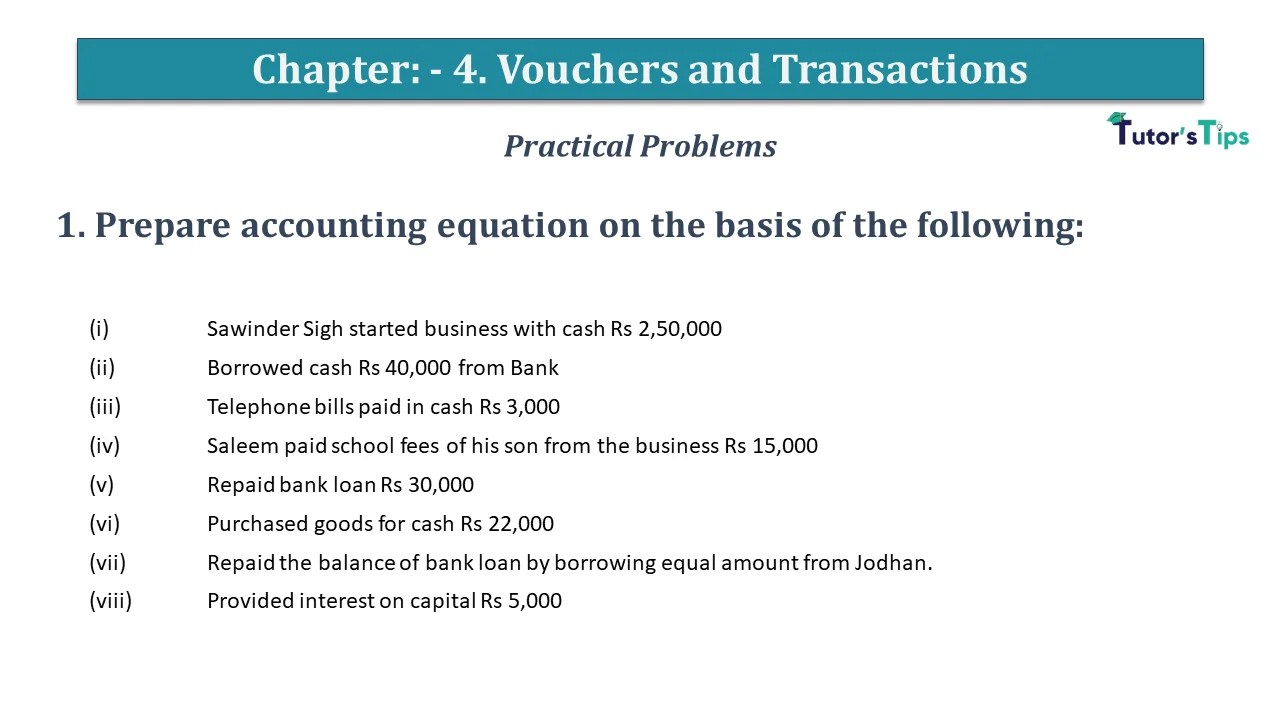Question No 1 Chapter No 4 1. Prepare accounting equation on the basis of the following:     (i)  Sawinder ...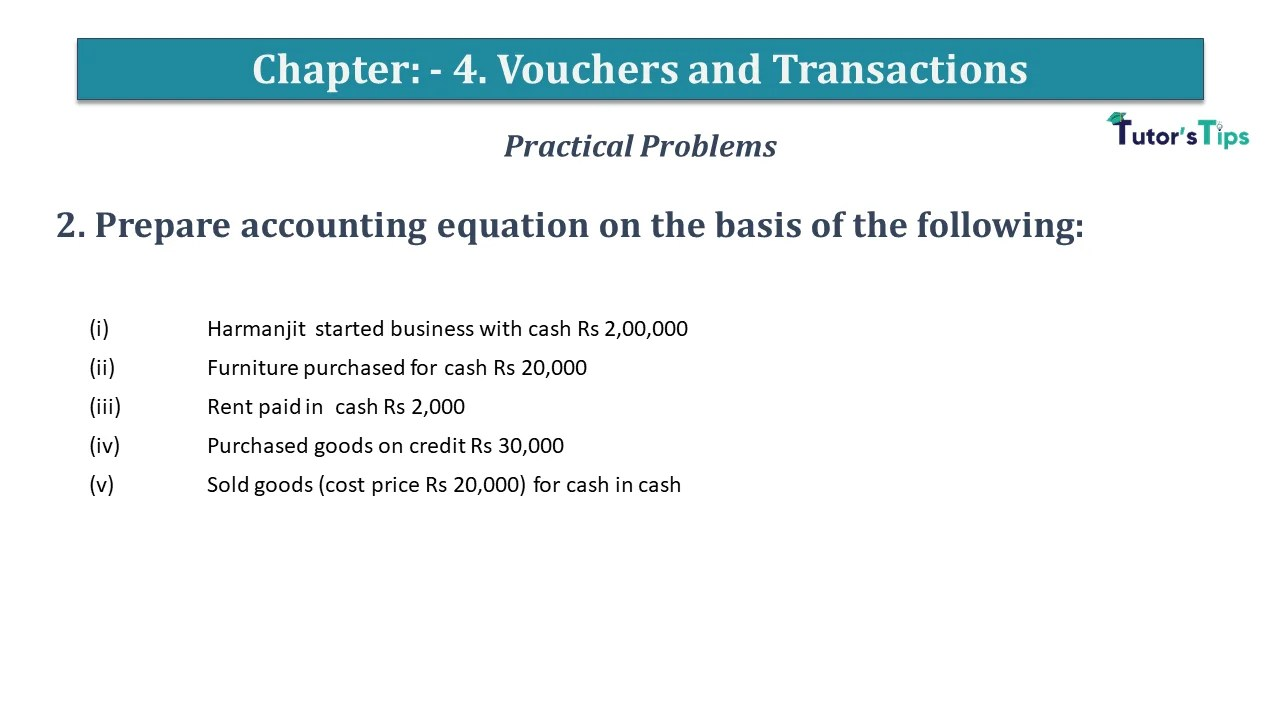Question No 2 Chapter No 4 2. Prepare accounting equation on the basis of the following: (i)  Harmanjit  started the ...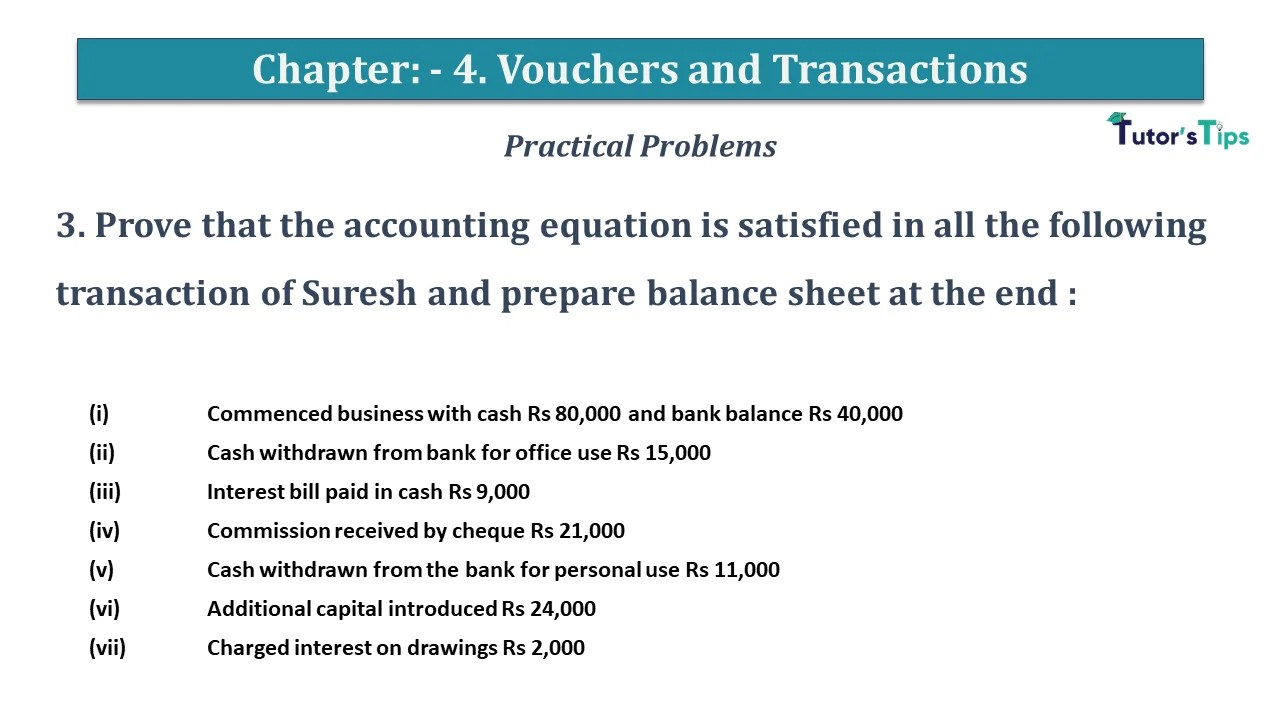Question No 3 Chapter No 4 3. Prove that the accounting equation is satisfied in all the following transaction of ...Question No 4 Chapter No 4 4. Show the effect of the following transaction on the accounting equations and prepare ...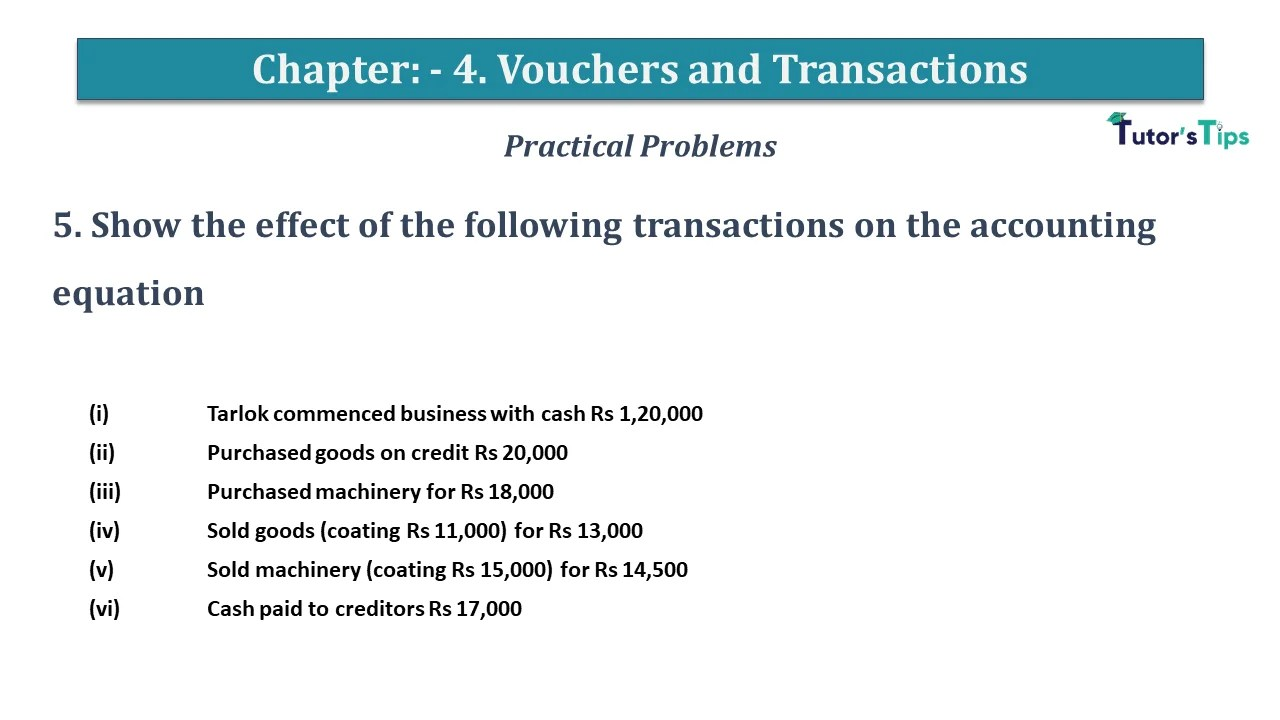Question No 5 Chapter No 4 5. Show the effect of the following transactions on the accounting equation     ...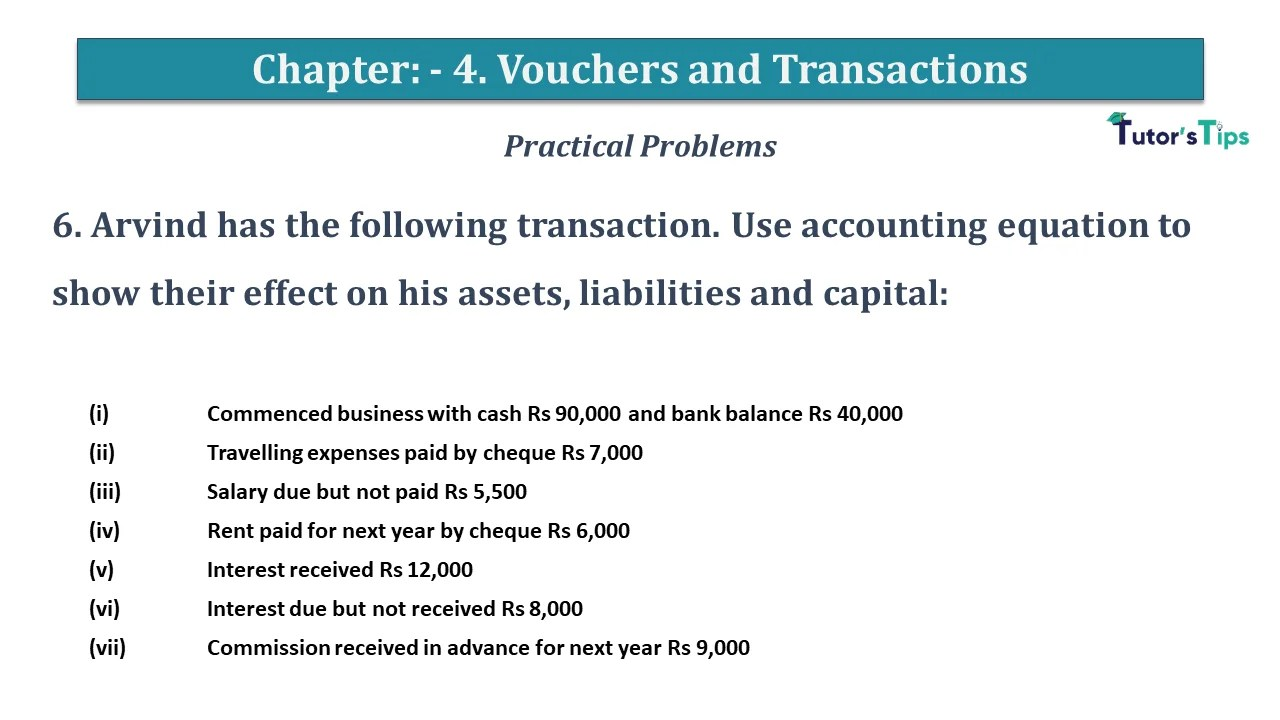Question No 6 Chapter No 4 6. Arvind has the following transaction. Use accounting equation to show their effect on ...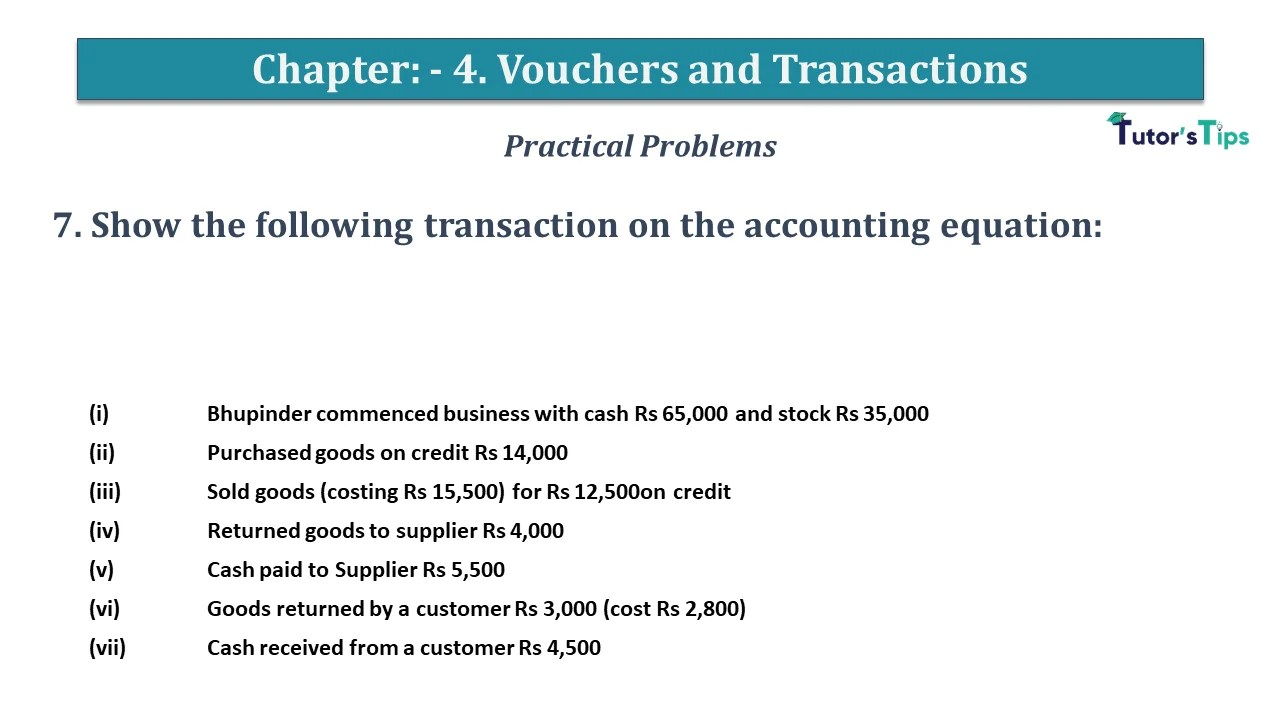Question No 7 Chapter No 4 7. Show the following transaction on the accounting equation: (i)  Bhupinder commenced business with ...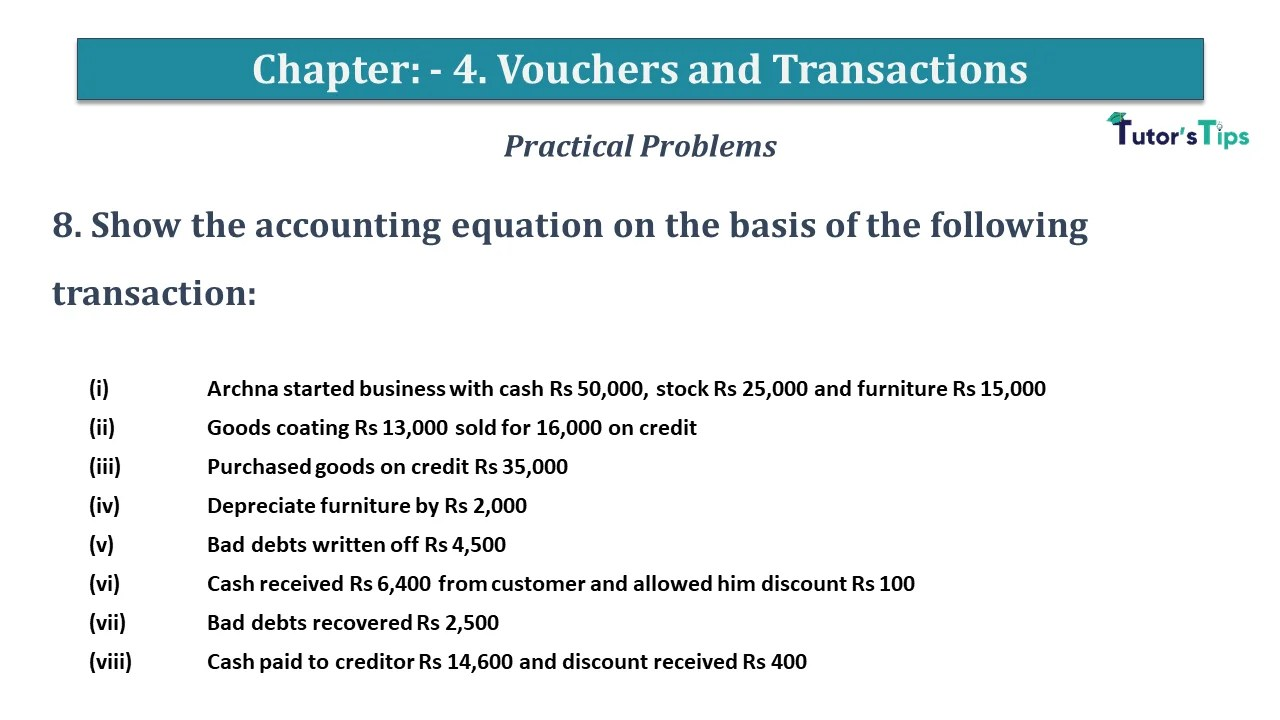Question No 8 Chapter No 4 8. Show the accounting equation on the basis of the following transaction: (i)  Archna ...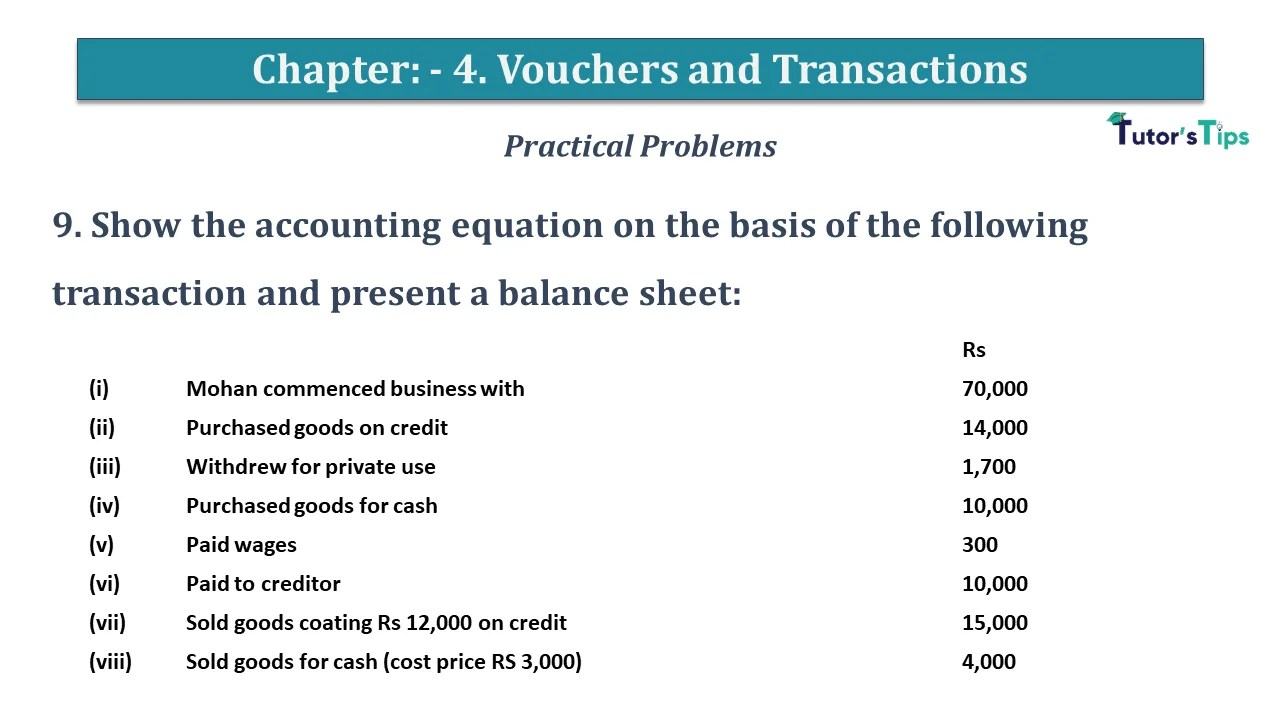Question No 9 Chapter No 4 9. Show the accounting equation on the basis of the following transaction and present ...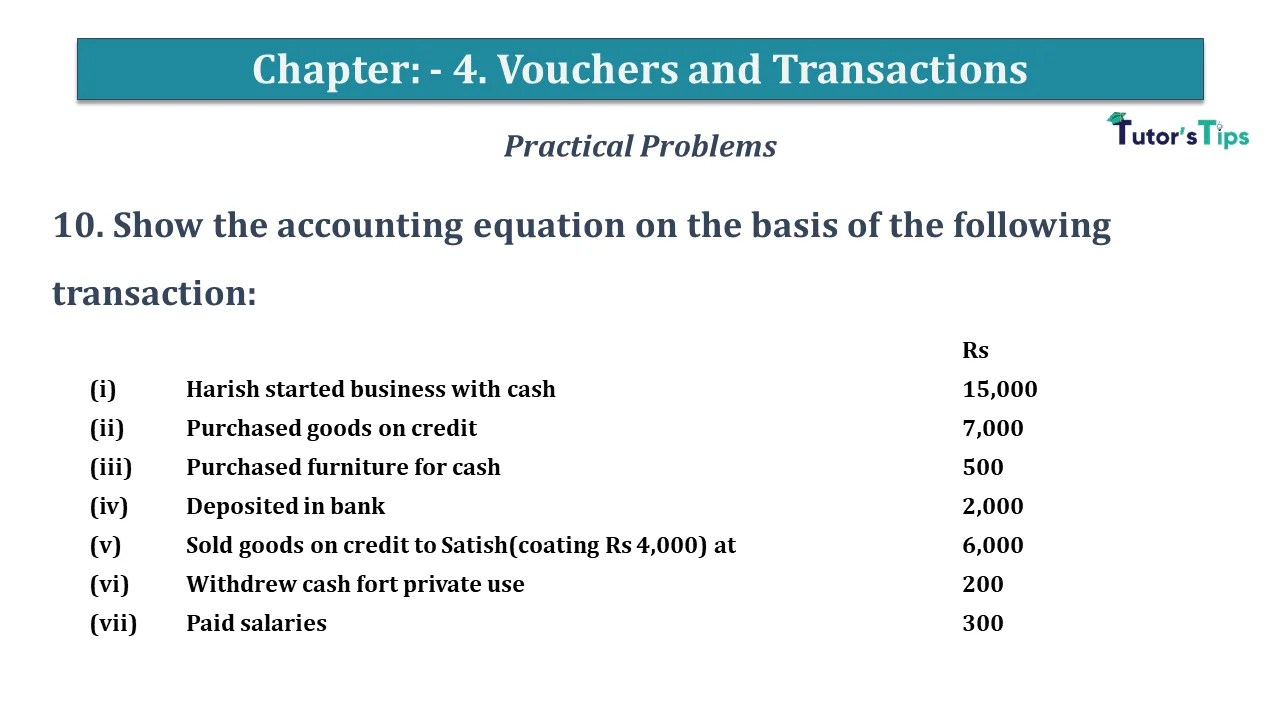Question No 10 Chapter No 4 10. Show the accounting equation on the basis of the following transaction:     ...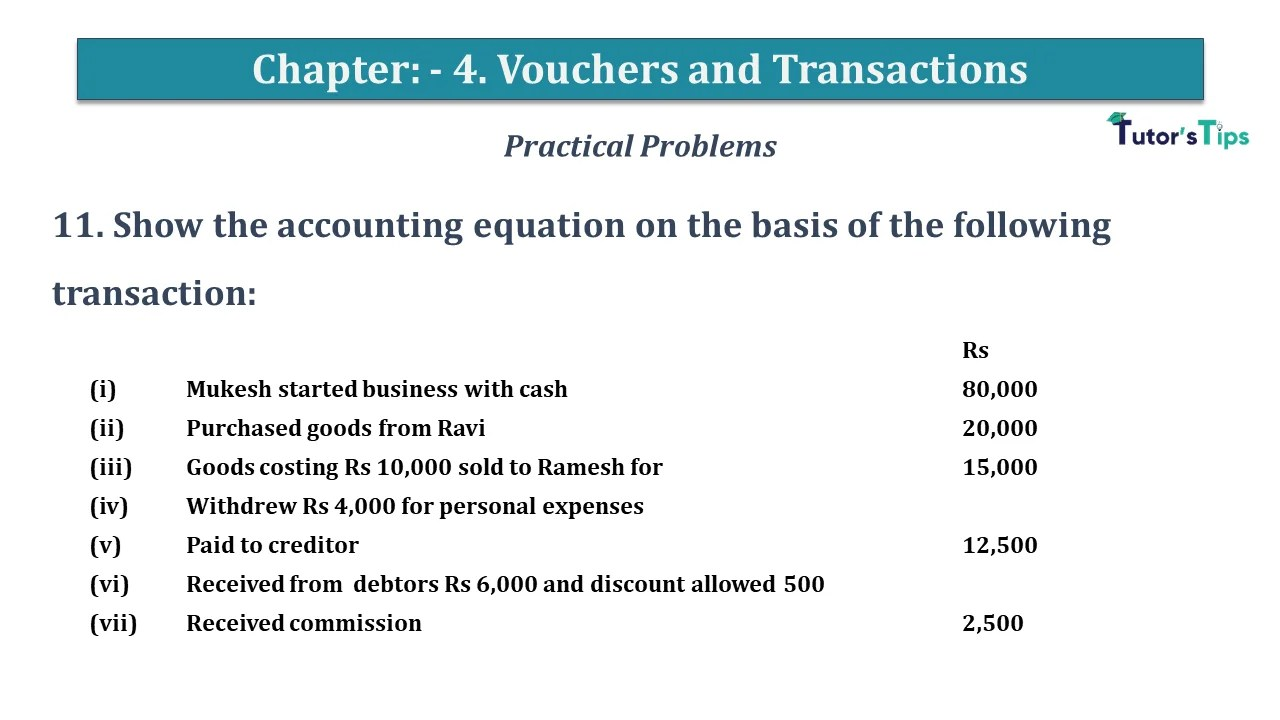Question No 11 Chapter No 4 11. Show the accounting equation on the basis of the following transaction:     ...Question No 12 Chapter No 4 12. Supriya had the following transaction. Use accounting to show their effect on his ...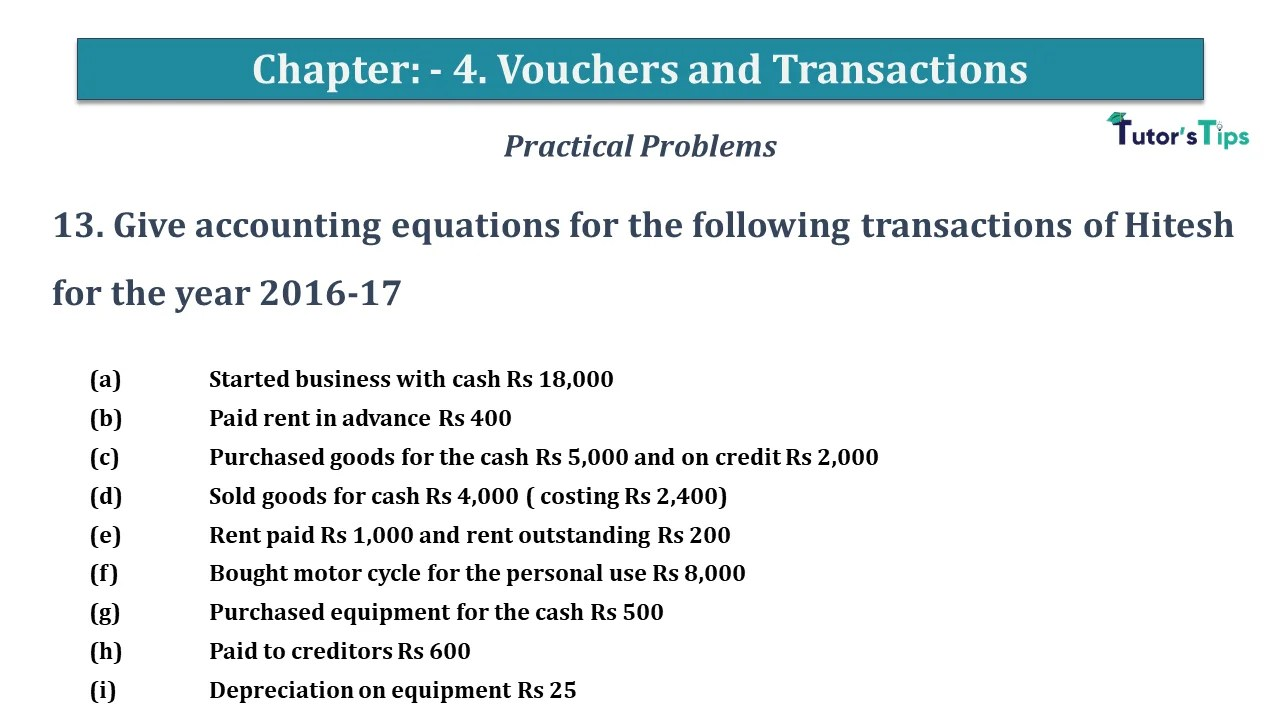Question No 13 Chapter No 4 13. Give accounting equations for the following transactions of Hitesh for the year 2016-17 ...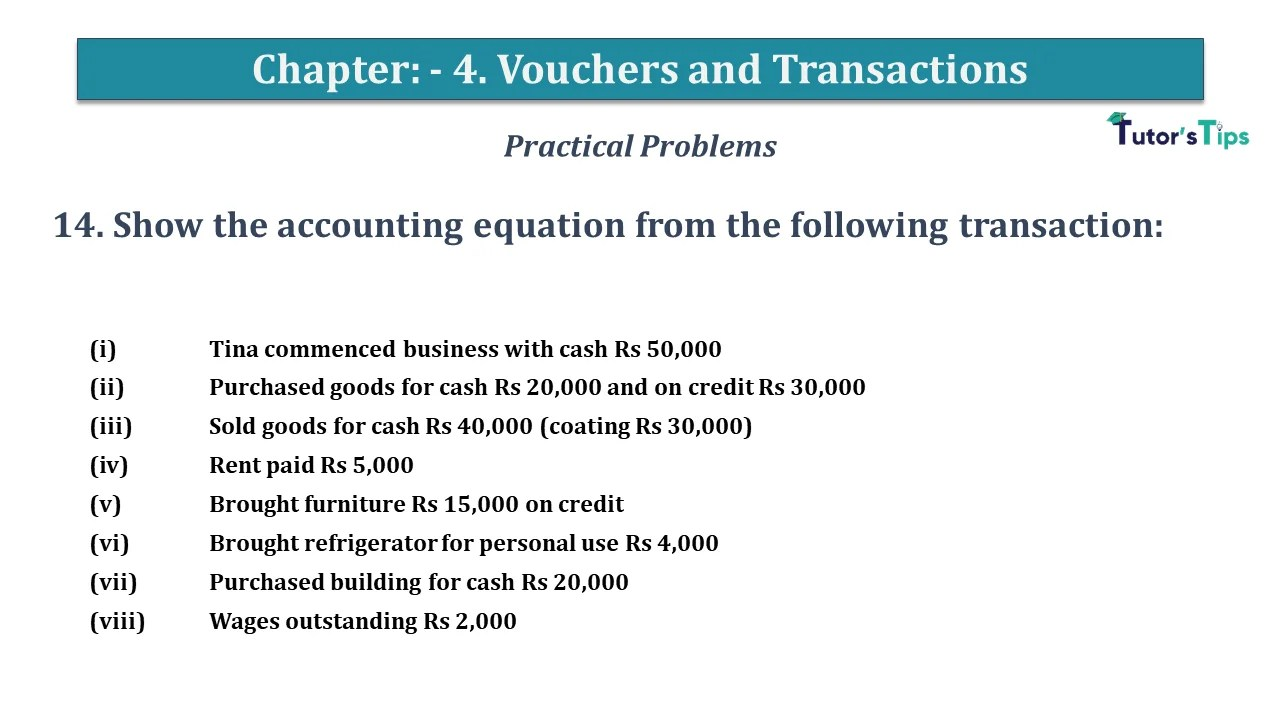Question No 14 Chapter No 4 14. Show the accounting equation from the following transaction: (i)  Tina commenced business with ...Question No 15 Chapter No 4 15. Show the accounting equation from the following transaction:     (i)  George started ...Question No 16 Chapter No 4 16. Show the effect of the following transaction on accounting equation:     (i)  ...Question No 17 Chapter No 4 17. Show the effect of the following transaction on accounting equation: (i)  Divedi stated ...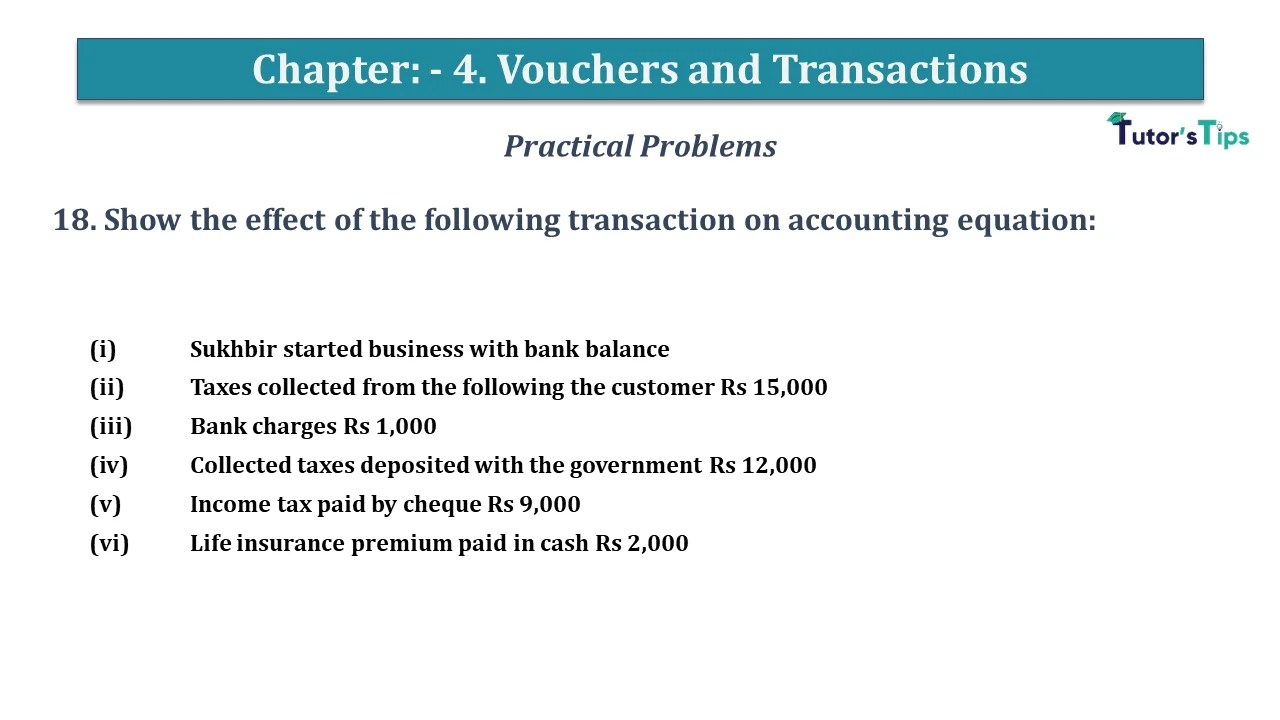Question No 18 Chapter No 4 18. Show the effect of the following transaction on accounting equation:     (i)  ...Question No 19 Chapter No 4 19. From the effect of the following transaction of Sumitra, prepare the accounting equation: ...Question No 20 Chapter No 4 20. From the following transaction of Madhu Bala, prepare the accounting equation:     ...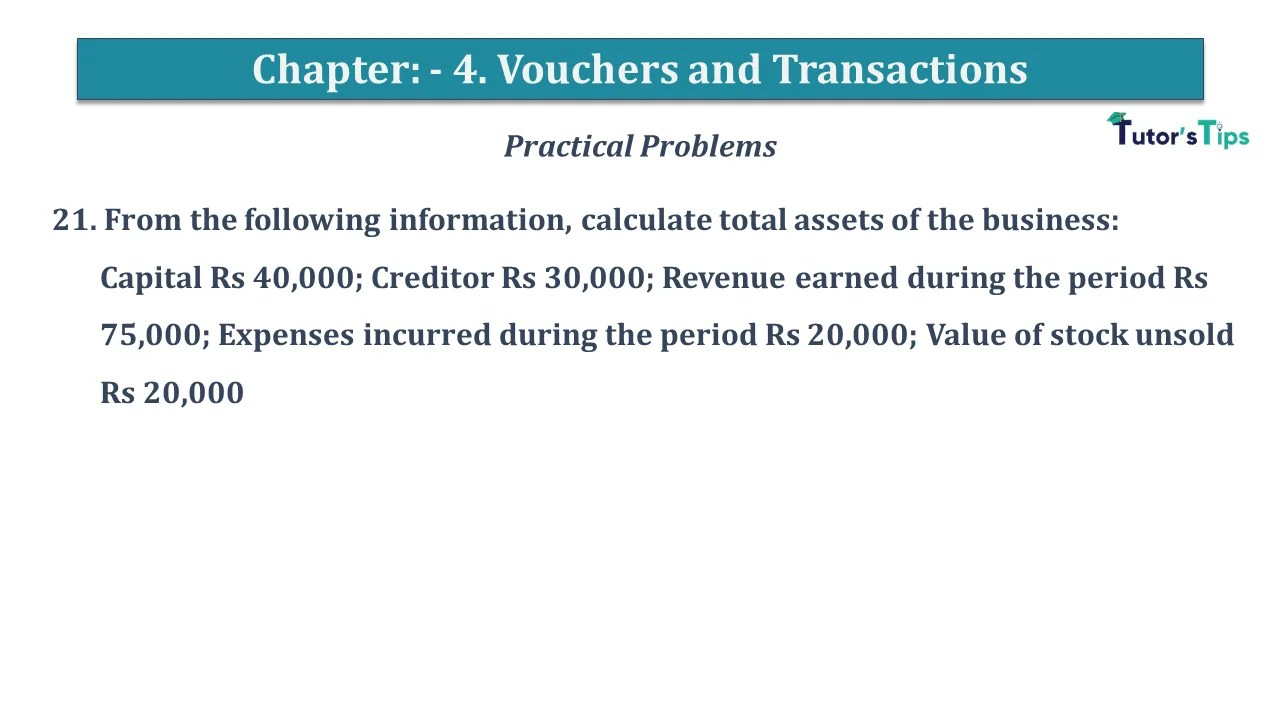Question No 21 Chapter No 4 21. From the following information, calculate total assets of the business:Capital Rs 40,000; Creditor ...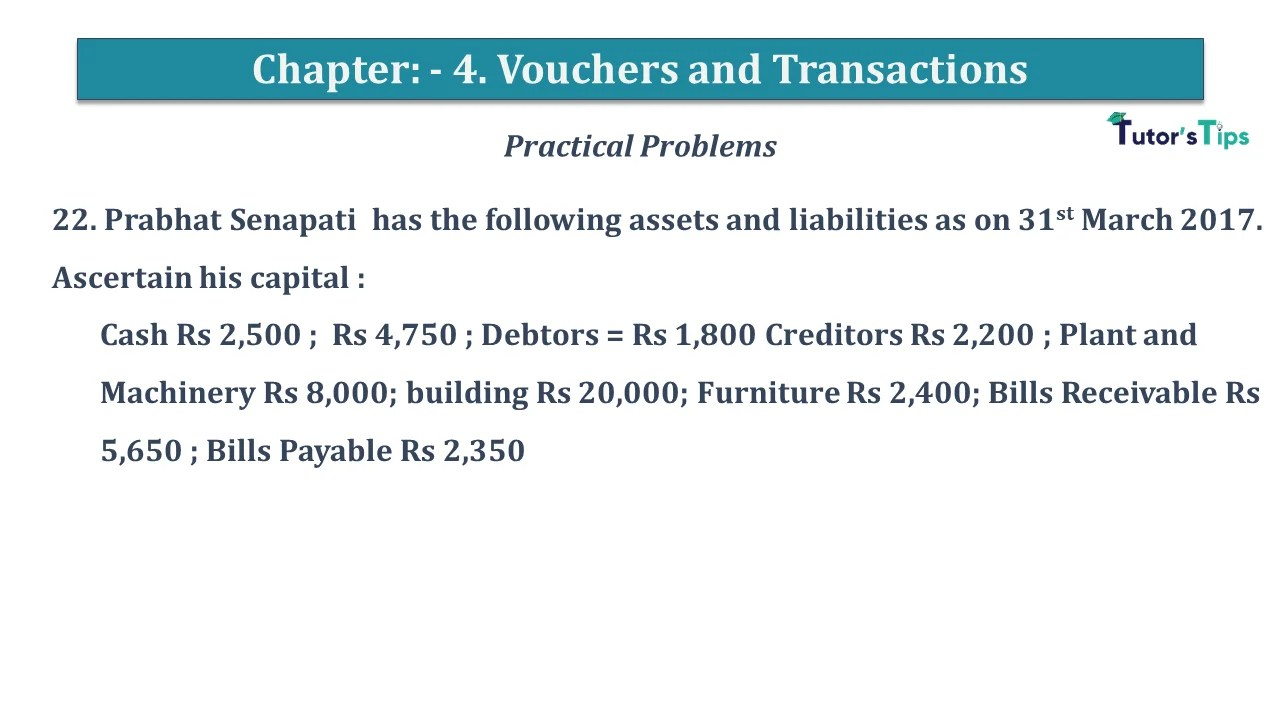Question No 22 Chapter No 4 22. Prabhat Senapati has the following assets and liabilities as on 31st March 2017 ...Question No 23 Chapter No 4 23. Rehman commenced business on 1st April 2018 with a capital of Rs 5,00,000 ...Question No 24 Chapter No 4 24. Parveen commenced business on 1st April 2018 with a capital of Rs 5,00,000 ...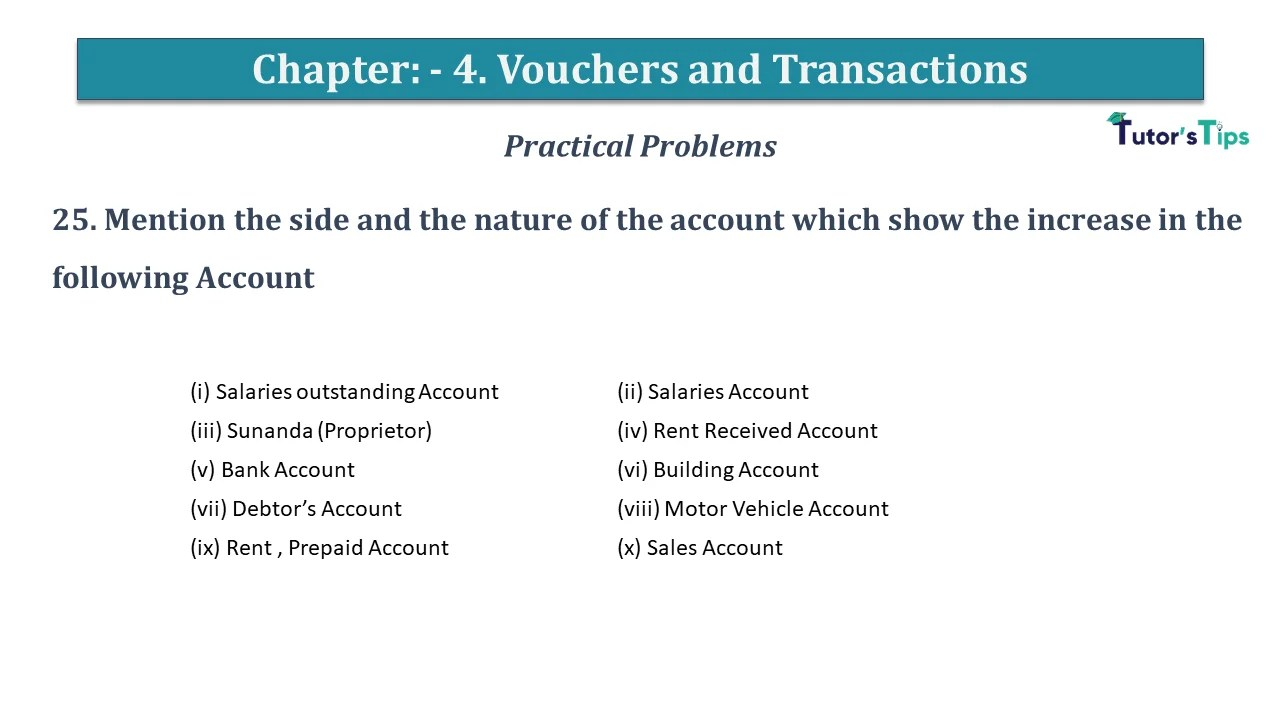Question No 25 Chapter No 4 25. Mention the side and the nature of the account which show the increase ...Question No 26 Chapter No 4 26. Mention the side and the nature of the account which show the decrease ...Question No 27 Chapter No 4 27. Analyse the following transactions using the rule of Debit and Credit under the ...Question No 28 Chapter No 4 28. Prepare “T” Shape account for the Motor Vehicle and write the following transaction ...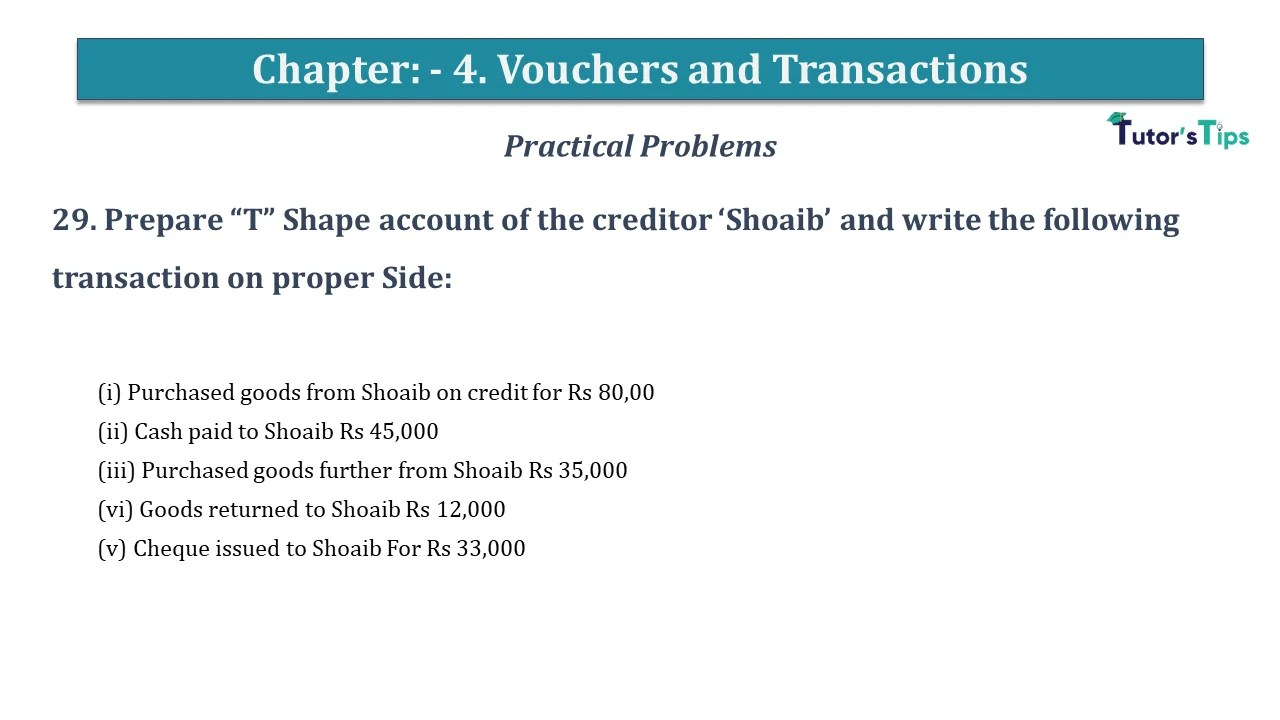Question No 29 Chapter No 4 29. Prepare “T” Shape account of the creditor ‘Shoaib’ and write the following transaction ...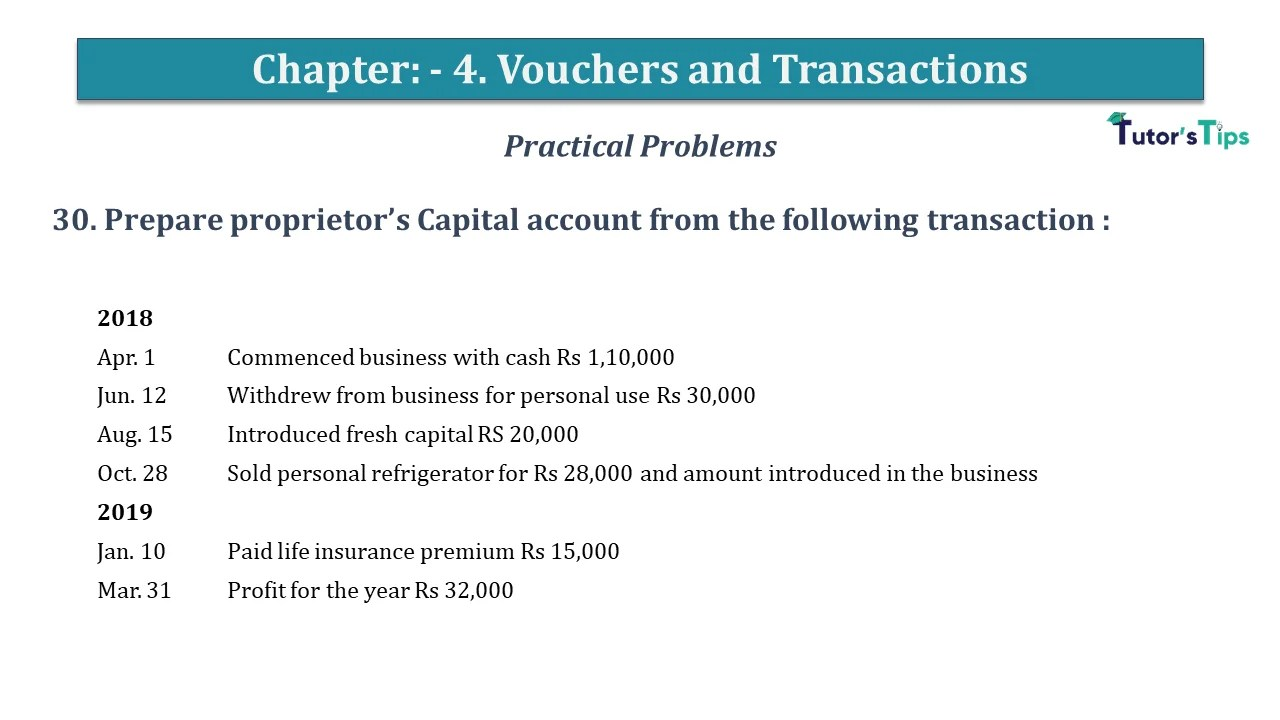Question No 30 Chapter No 4 30. Prepare proprietor’s Capital account from the following transaction : 2018   Apr. 1 ...

error: Content is protected !!